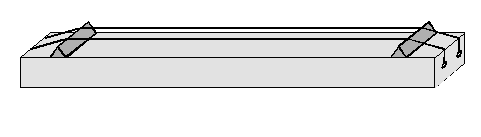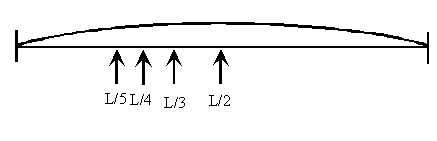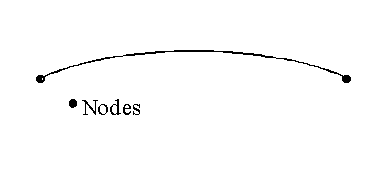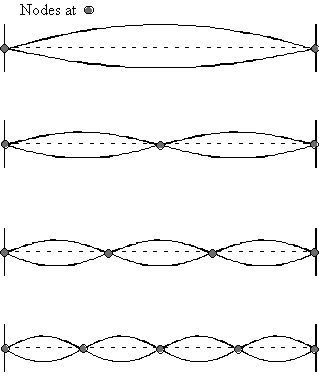3.3 Vibrating Strings

Pythagoras started his studies of musical scales by noting that two strings whose lengths formed certain ratios sounded pleasing when played together. How was this done in practice? One can make a two stringed instrument and adjust the tension on the strings so that they have the same pitch (an interval of a unison):On most string instruments like this, the pitch is changed as one plays, by placing a finger on the string and pressing down hard. This restricts the length of string that can vibrate, which then raises the pitch. If you press String 2 half way along its length (at point A) it will vibrate like a string half of its length and its frequency will be a factor of 2 higher. This new pitch will then form an octave with respect to String 1.

To form a Perfect Fifth between the two strings, we want the frequency of String 2 to be a factor of 3/2 higher, or its length should be a factor of 2/3 shorter. So, we want to place our finger 2/3 the distance from one end, or 1/3 the distance from the other end. This puts us at point B. As long as the longer piece of the string is vibrating, the pitch will now be a Perfect Fifth higher than String 1.

To form a Perfect Fourth, the frequency of String 2 should be 4/3 higher, or the length 3/4 shorter. Thus, we can place our finger 3/4 the distance from one end, or 1/4 the distance from the other end. Again, we assume that the longer piece of the string vibrates.

All in all, we find that there are special points on a vibrating string such that when we press the string at those points the string vibrates at a frequency that forms a pleasing interval with respect to the identical string.If the string has a length of L, these special points occur at a distance L/n from one end of the string, where n is an integer. We could also say that they occur at a distance (n-1)L/n from the other end of the string. As it turns out, these points at L/n are special for another reason, as well. One that Pythagoras most likely did not realize himself!

Rather than press down hard with our finger, we will just very lightly touch the string at some point and pluck the string with our other hand. Usually, when we do this no good sound is produced, even though the whole string can vibrate. However, at certain points, a nice tone is produced with a much higher frequency than the original string. Moreover, the points where a nice tone is made are exactly the same points that we found above, namely a distance of L/n from one end of the string. In the case where we press down on the string, we essentially just formed a somewhat shorter string, but nothing else changed. However, when we just touched the string, the whole string could vibrate, yet it was producing a new pitch. In fact, after we pluck the string, we can remove our finger, and the string continues to vibrate at the new frequency! This is something new – it means that a string can vibrate at more than one frequency, but only certain new frequencies. The basic, or lowest frequency at which a string can vibrate is called the fundamental. In fact, the lowest frequency that anything can vibrate at is called its fundamental. All other frequencies that a string can produce are called overtones. Pythagoras based all of his work on the fundamental frequency of a string and he probably did not know that a string also has overtones.

Although we now know that a string can vibrate at more than one frequency, it cannot vibrate at any arbitrary frequency. So, the question now is: what frequencies can a string vibrate at? Before we try to answer this question, it is useful to consider exactly what a string looks like while it is vibrating. We know that the endpoints of a string do not move, as they are the places where the string is attached to the instrument. A point on a string that does not move is called a node. All strings have nodes at each end, but they can have additional nodes along the string. Here is what a simple string looks like with just nodes at the ends:Figure 1 - Simple string with nodes at the ends

What happens if we try to add another node right in the middle of the string?Here, notice that each part of the string is exactly half as long. The tension in the string and its composition has not changed, but a string half as long has a vibrational frequency that is twice as high. So, if the simple string has a frequency of f, the string with an extra node in the middle has a vibrational frequency of 2f.

What happens if the node is not exactly at the center of the string?Now the string is divided into two unequal sections. Each section would behave as a separate string, but since the lengths of the strings are different, their vibrational frequencies would be different. In this case, the whole shape of the string would not repeat with a single frequency. So, if only one node is added, it must be at the center of the string. In general, any number of nodes can be added to a string as long as each section of the string has the same frequency. In other words, each section of the string must have the same length.Each of these different patterns of nodes along the string is referred to as a mode of vibration, each with its own vibrational frequency. To determine the frequency, we just have to figure out the frequency of the shortest section between two nodes. For example, if the string has two extra nodes, the shortest section of the string has a length of L/3. Since the frequency of vibration is inversely proportional to the length of the string, a string with a length of L/3 will have a frequency of 3x(fundamental frequency), or 3f. In general, the short section of the string has a length of L/n, if there are (n-1) extra nodes. So, the overtone would be nf. The whole series is then 2f, 3f, 4f, 5f, …

Nodes are the points on the string that do not move as the string vibrates. So, the points on the string that move the most are called antinodes. The antinodes are exactly half way between the nodes.

An interesting question arises from this analysis: how exact are the integers? In other words, if the fundamental has a frequency of 350 Hz, is the second harmonic exactly 700 Hz? In fact, the harmonics are nearly perfect integers. The concepts of precision and accuracy are rather hard to define, but there is a simple way to see how accurate the harmonics are. Imagine trying to pick a point exactly one third of the way from one end of the string without using a ruler – just by eye. You probably wont be very accurate. Now imagine trying to find a point one third of the distance from the end by listening for a clear harmonic. When you find this point, you will have divided the string into three parts far more accurately than you did by eye. This shows that there are different ways to measure things and often, if you base the measurement on frequencies, the measurement will be the most accurate. This is the concept of spectroscopy.

The set of frequencies at which a string or any system can vibrate at is called an overtone series. There are many systems, such as a string, which have a simple overtones series, in which all of the overtones are integer multiples of the fundamental. This is called a harmonic series. Finally, it sometimes happens that a system vibrates only at harmonics of the fundamental, but not all harmonics are possible, for example, the series f, 3f, 5f, … This is called a partial harmonic series. I.e. this is a harmonic series with some of the harmonics missing.

One final note for this section: in modern physics, this idea that a system has only special, very specific, modes of vibration, each with a particular vibrational frequency is called "quantization". Each mode of vibration is called a quantum state and the properties of these states are understood through quantum mechanics. It was the realization that objects like electrons and protons are actually waves and had modes of vibration that led to the rapid development of modern physics in the 1900’s. Quantum mechanics made possible almost all modern technologies in one way or another. For example, computers, nuclear reactors, lasers, magnetic resonance imaging (MRI), are all based on quantum mechanics.